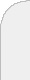inicioir al DIIbuscarpropagation of photons in a scattering media and the inverse We consider the Fokker-Planck equation as the model for the propagation of photons in a media with highly peaked forward scattering. Then we look at the inverse problem of reconstructing the optical parameters of the equation from boundary measurements. We will present results on how to approximate the solution of the Fokker-Planck equation using a paraxial approximation and then comparing trajectories of stochastic processes. An explicit solution for the paraxial approximation is known, and therefore we can  approximately reconstruct the Fokker-Planck optical parameters for the case of small scattering.